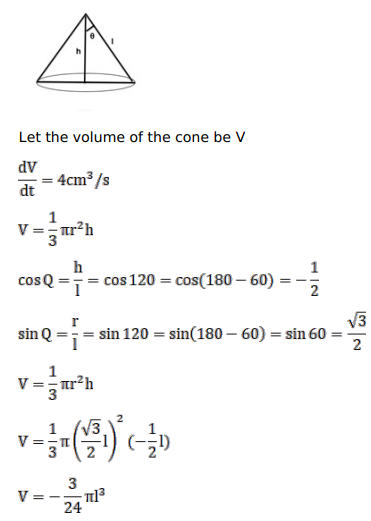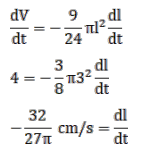# Water is dripping through a tiny hole at the vertex in the bottom of a conical funnel at a uniform rate of 4

Question:

Water is dripping through a tiny hole at the vertex in the bottom of a conical funnel at a uniform rate of 4 $\mathrm{cm}^{3} / \mathrm{s}$. When the slant height of the water is $3 \mathrm{~cm}$, find the rate of decrease of the slant height of the water, given that the vertical angle of the funnel is $120^{\circ}$.

Solution: